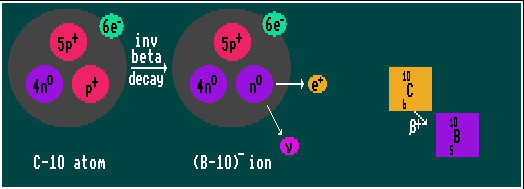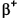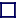### INVERSE BETA DECAY

INVERSE BETA DECAY (BETA+) (POSITRON EMISSION) / ELECTRON CAPTURE
Inverse beta decay (+ decay) can occur when (artificially produced) nuclides have an excess of protons, and one of the protons changes into a neutron via the weak force emitting a positron and a neutrino. Note that a free proton cannot decay into a neutron because it has less mass; but inside a nucleus, a bound proton may be in a higher energy state than a bound neutron, and inverse beta decay can proceed. An alternative and competing process to inverse beta decay is Electron Capture where an internal s-electron is captured by a proton changing it into a neutron and emitting a neutrino. Because the electron is absorbed into the nucleus, no beta radiation is emitted.

For light nuclei, inverse beta decay is favoured, but for heavier nuclei, electron capture is overwhelmingly predominant. Either way, the Atomic Number decreases by one but the Atomic Weight remains constant. However, in the case of inverse beta decay, a positron is emitted, leaving the parent atom now negatively charged with one extra electron, which it quickly loses. The spare electron can remain with the atom, producing a negative ion or it can escape. The positron, meanwhile, may mutually annihilate with an electron producing gamma rays of 1MeV energy. In cases where the atomic mass difference between the decaying atom and the product atom is less than two electron masses, then inverse beta decay cannot occur, but electron capture can, providing the product is lighter than the parent. In inverse beta decay, the positively charged positron emitted from the nucleus is repelled by the positively charged protons within the nucleus, and thus both the probability of decay and the particle energies are increased. (The converse is true for beta decay). Also, as atomic number (Z) increases, so does the magnitude of this effect. In inverse beta decay, the decay energy minus 1.022MeV (twice the electron rest mass) is available. The emerging relativistic positron is barely affected by the surrounding and diffuse electron cloud.

Because inverse beta decay involves the weak-force carrying particle (the W+) and because the weak force violates parity conservation, inverse beta decay always produces right-handed positrons and left-handed neutrinos, never the other way around. Conversely, beta decay involves the W- particle, and always produces left-handed electrons and right-handed anti-neutrinos. See 'Higgs and Left-handed [and right-handed? neutrinos'].

Shown is carbon-10 decaying by inverse beta decay into the stable boron-10.